Ex 1.1

Chapter 1 Class 8 Rational Numbers
Serial order wise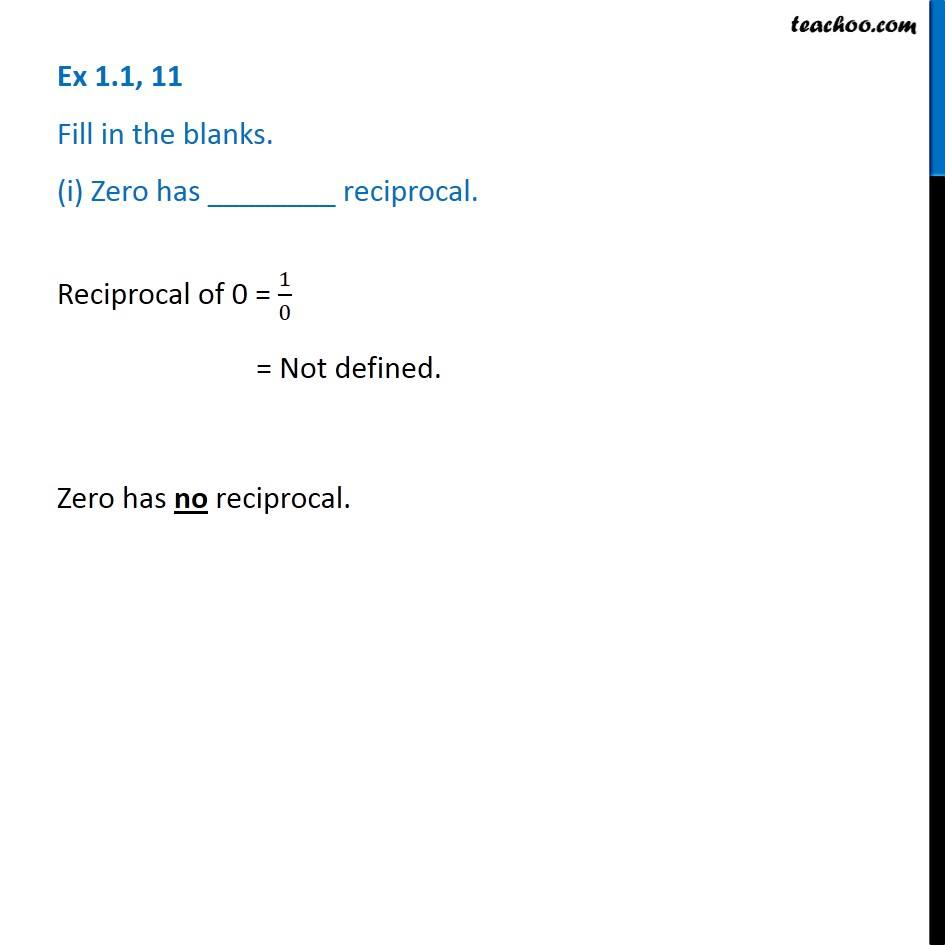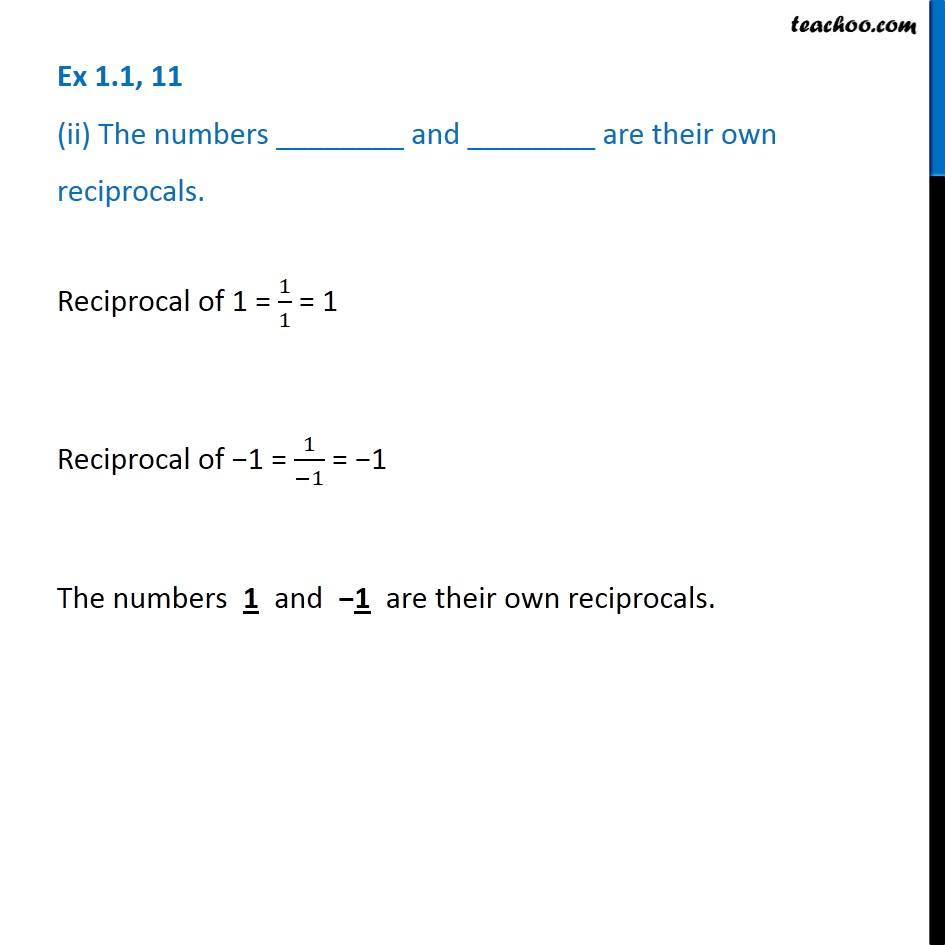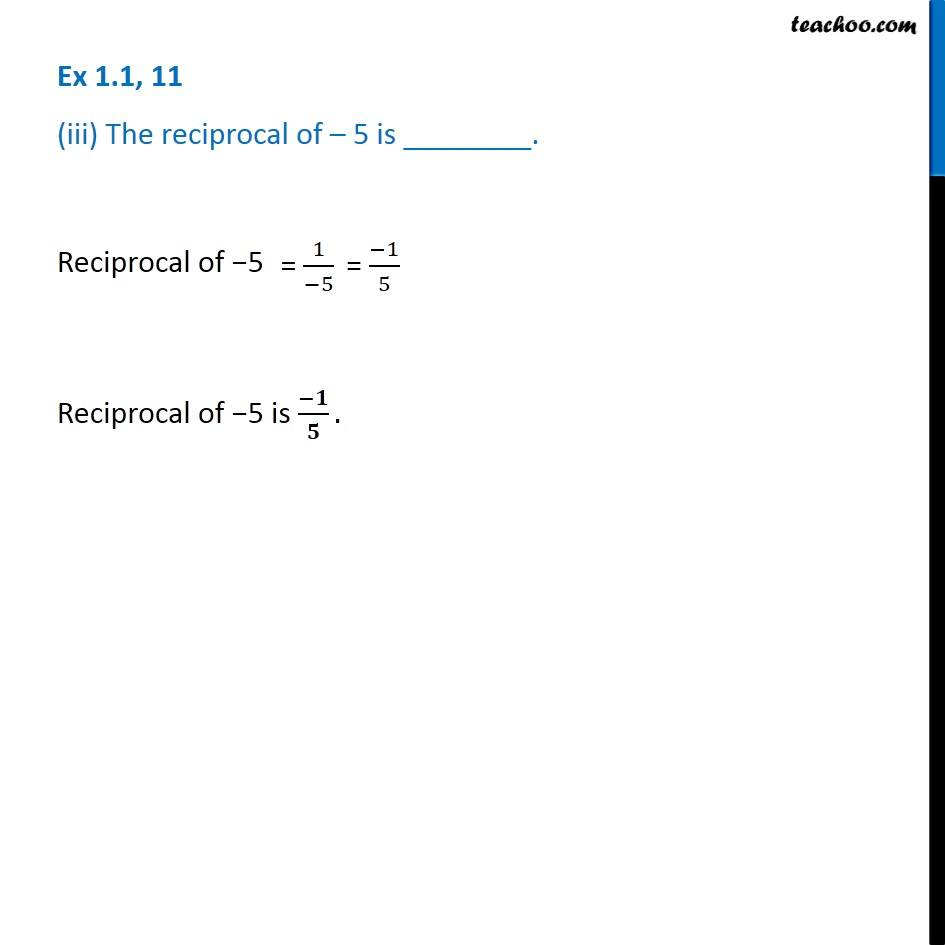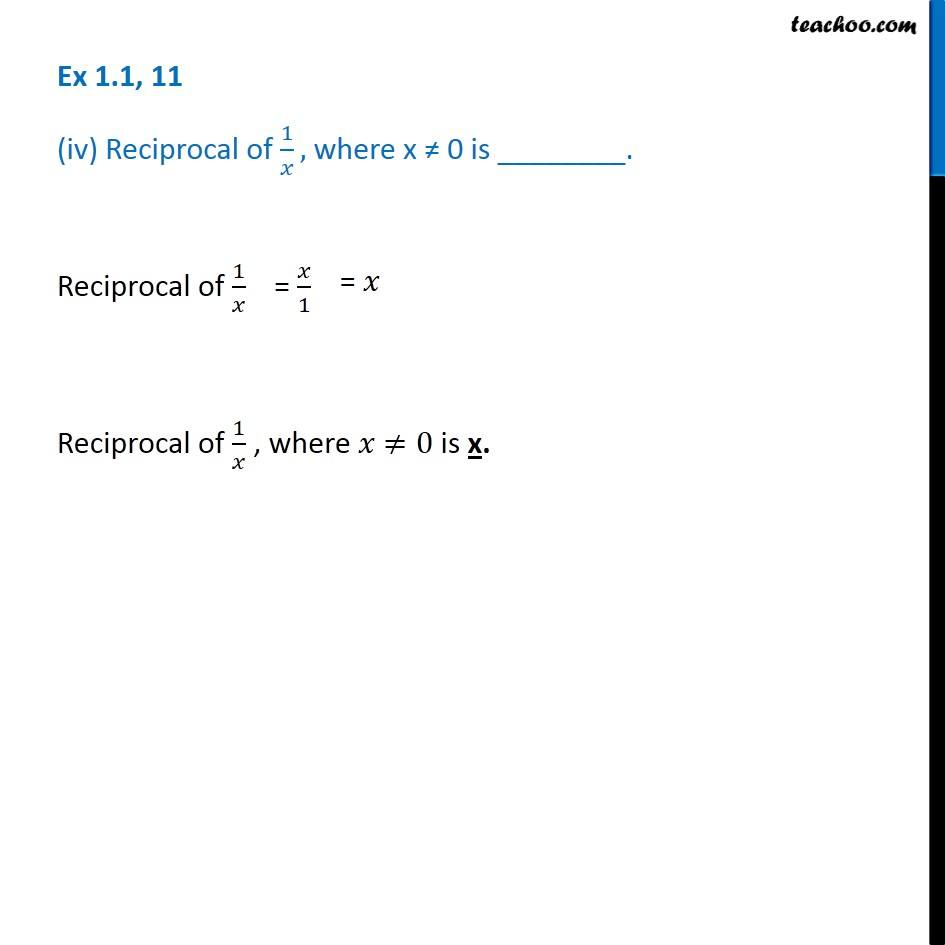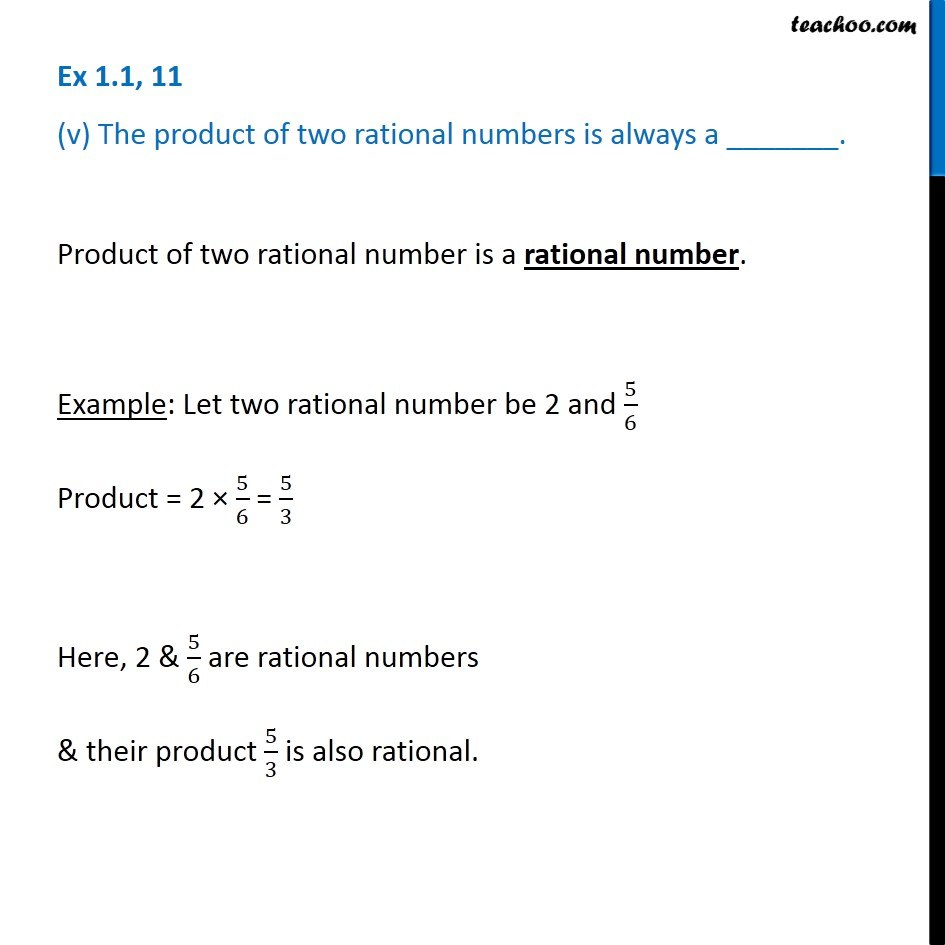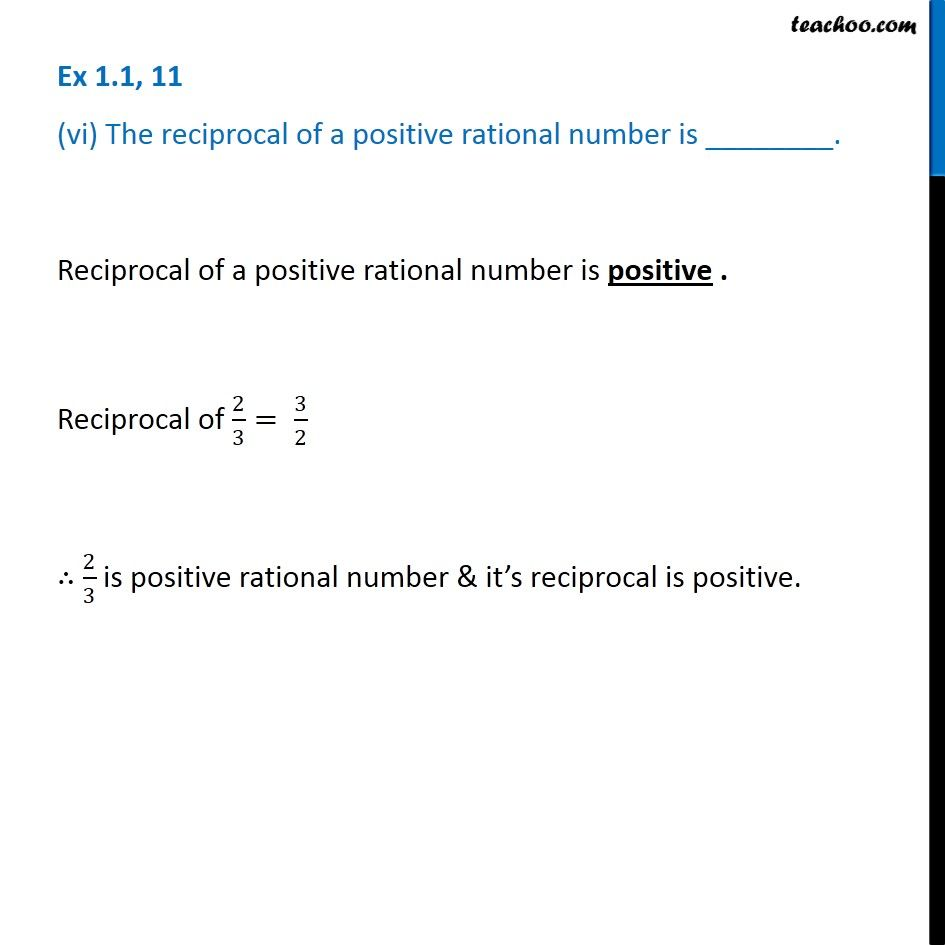Get live Maths 1-on-1 Classs - Class 6 to 12

### Transcript

Ex 1.1, 11 Fill in the blanks. (i) Zero has ________ reciprocal. Reciprocal of 0 = 1/0 = Not defined. Zero has no reciprocal. Ex 1.1, 11 (ii) The numbers ________ and ________ are their own reciprocals. Reciprocal of 1 = 1/1 = 1 Reciprocal of 1 = 1/( 1) = 1 The numbers 1 and 1 are their own reciprocals. Ex 1.1, 11 (iii) The reciprocal of 5 is ________. Reciprocal of 5 Reciprocal of 5 is ( )/ . Ex 1.1, 11 (iv) Reciprocal of 1/ , where x 0 is ________. Reciprocal of 1/ Reciprocal of 1/ , where 0 is x. Ex 1.1, 11 (v) The product of two rational numbers is always a _______. Product of two rational number is a rational number. Example: Let two rational number be 2 and 5/6 Product = 2 5/6 = 5/3 Here, 2 & 5/6 are rational numbers & their product 5/3 is also rational. Ex 1.1, 11 (vi) The reciprocal of a positive rational number is ________. Reciprocal of a positive rational number is positive . Reciprocal of 2/3= 3/2 2/3 is positive rational number & it s reciprocal is positive.